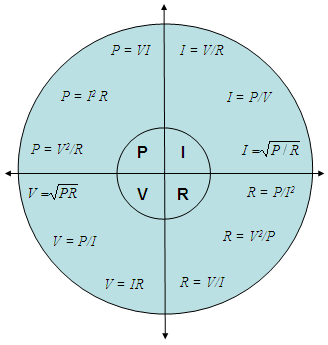# Ohm’s law Calculator

 The Ohm’s law (after Georg Ohm) defines the relationship between voltage (V), current (I) and resistance (R). Power (P) relationships appear as a logic consequence of the others. One ohm is the resistance value through which one volt will produce a current of one ampere. The Ohm’s formula is   V = IR.

Voltage (V) is the difference in electrical potential between two points in an electronic circuit, and it is measured in volts (V).

Current (I) is a flow of electrons on a conductor, and it is measured in amperes (A).

Resistance (R) determines how much current will flow through an electrical device. Resistors are used to control or limit voltage and current levels. A high resistance will allow a small current to flow. A low resistance will allow a larger current to flow. Resistors are measured in ohms.

Power (P) is the voltage multiplied by the current, and it’s measured in watts (W).Ohm’s law Pie Chart

### Electrical Calculations

Let’s say that a 12V car battery is connected to a 3 ohm light bulb. What is the voltage measured at the light bulb?

We can use our calculator and enter

V = 12.0 V
R = 3.0 ohms

We get

I = 4.0 A

### Parallel Resistors

Our calculator can also be used to find out the equivalent resistor of up to three resistors in parallel. For example, three 20-ohm parallel resistors are connected across a 120 V power source. What is the current of this circuit?

We use the section on parallel resistors and enter

R1 = 20 ohms
R2 = 20 ohms
R3 = 20 ohms

we learn that the equivalent resistor is Req = 6.7 ohms.

Now, we use the formula I = V/R and enter the known values of voltage and resistance

V =   120 V
R =   6.7 ohms

and we discover that the total current flowing is I = 17.91 amperes.